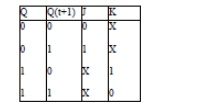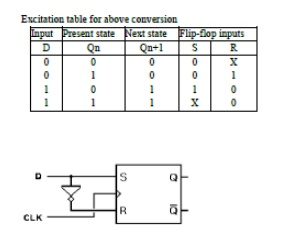Home | | Digital Logic Circuits | Important Short Questions and Answers: Synchronous Sequential Circuits

# Important Short Questions and Answers: Synchronous Sequential Circuits

Digital Logic Circuits - Synchronous Sequential Circuits - Important Short Questions and Answers: Synchronous Sequential Circuits

SYNCHRONOUS SEQUENTIAL CIRCUITS

1. What are shift register counters? List two widely used shift register counters.

Solution:

If the output of a shift register is fed back to the input. a ring counter results. The data pattern contained within the shift register will recirculate as long as clock pulses are applied.

2. Why is FlipFlop also known as Latch?

Solution:

The difference between a latch and a flip-flop is that a latch does not have a clock signal, whereas a flip-flop always does. Latch is a level sensitive device while flip-flop is an edge sensitive device. Latch is sensitive to glitches on enable pin, whereas flip-flop is immune to that.

3. Compare Moore and Mealy Machine.4. Obtain the excitation table of D-FlipFlop4. What are state diagram and state tables?

Solution:

The time sequence of inputs, outputs and flip-flop states may be enumerated in a state table and the information available in a state table may be represented graphically called a state diagram. For the design of sequential counters we have to relate present states and next states. The table, which represents the relationship between present states and next states, is called state table.

5. Give the excitation table for JK FlipFlop

Solution:6. Draw the logic diagram for T FlipFlop

Solution:7. How many flip-flops are required to design a mod-7 up-down counter?

Solution:

Flip Flops required are 2n >= N where N=7

2n >= 7 n = Number of Flip Flops.

n = 3 Hence 3 Flip Flops are required

8. What is a sequential circuit?

Solution:

The logic circuits whose outputs at any instant of time depend not only on the present inputs but also on the past outputs are called sequential circuits. Example : flip-flops.

10.   Draw the logic diagram of SR flip-flop.

Solution:11. Draw the timing diagram of 4-bit ring counter.12. Write the characteristic equation of JK flip-flop.

Solution:

Q(t + 1) = JQ' + K'Q13.State a limitation of SR flip-flop.

Solution:

The last input condition in SR flip-flop is S=1 and R=1. This Condition will produce 0 at the output of both the NOR gate. Hence Qn+1=0 and Q'n+1= 0. This condition violates the fact that the outputs Qn+1 and Q'n+1 are the complements of each other. In normal operation, this condition must be avoided by making sure that 1s are not a applied to both inputs simultaneously.

14. Convert a D flip-flop into T flip-flop.15. What is the race- around condition?

Solution:

In JK FF output is fedback to the input and therefore change in the output results in change in the input.If the FF is level trigged, in the positive half of the clock pulse if j and k are both high then output toggles continuously. This condition is known as race around condition.

Study Material, Lecturing Notes, Assignment, Reference, Wiki description explanation, brief detail
Digital Logic Circuits : Synchronous Sequential Circuits : Important Short Questions and Answers: Synchronous Sequential Circuits |